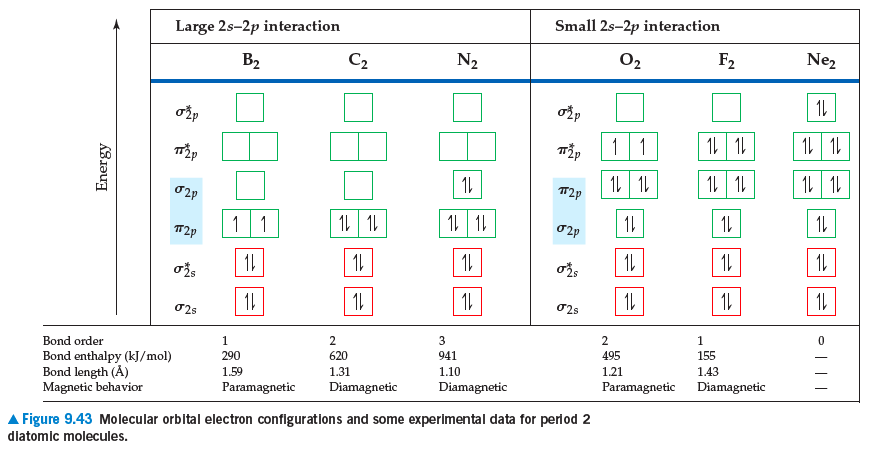# Problem: You may want to reference(Pages 371 - 382)Section 9.8 while completing this problem.If we assume that the energy-level diagrams for homonuclear diatomic molecules shown in Figure 9.42 in the textbook can be applied to heteronuclear diatomic molecules and ions, predict the bond order and magnetic behavior of each: (a) CO+, (b) NO–, (c) OF+, (d) NeF+.

⚠️Our tutors found the solution shown to be helpful for the problem you're searching for. We don't have the exact solution yet.

###### Problem Details

You may want to reference(Pages 371 - 382)Section 9.8 while completing this problem.

If we assume that the energy-level diagrams for homonuclear diatomic molecules shown in Figure 9.42 in the textbook can be applied to heteronuclear diatomic molecules and ions, predict the bond order and magnetic behavior of each: (a) CO+, (b) NO, (c) OF+, (d) NeF+.